Courses

# Past Year Questions: Structural Analysis Notes | EduRev

## Mechanical Engineering : Past Year Questions: Structural Analysis Notes | EduRev

The document Past Year Questions: Structural Analysis Notes | EduRev is a part of the Mechanical Engineering Course Engineering Mechanics.
All you need of Mechanical Engineering at this link: Mechanical Engineering

Question 1:Two identical trusses support a load of 100 N as shown in the figure. The length of each truss is 1.0 m, cross-sectional area is 200 mm2; Young's modulus E = 200 GPa. The force in the truss AB (in N) is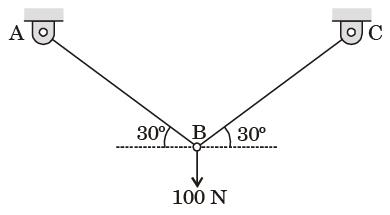Question 2:A two member truss PQR is supporting a load W. The axial forces in members PQ and QR are respectively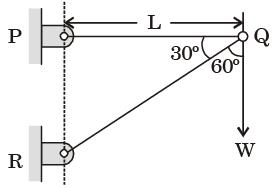Question 3:For the truss shown in the figure, the magnitude of the force (in kN) in the member SR is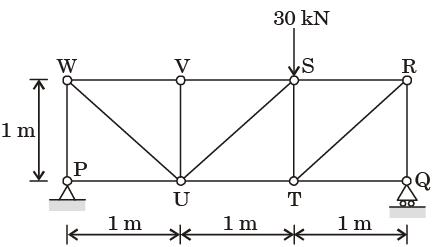Question 4:For the truss shown in figure, the magnitude of the force in member PR and the support reaction at R are respectively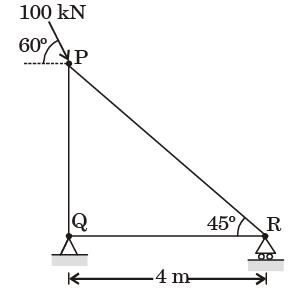Question 5:For the truss shown in the figure, the forces F1 and F2 are 9 kN and 3 kN, respectively. The force (in kN) in the member QS is (All dimensions are in m)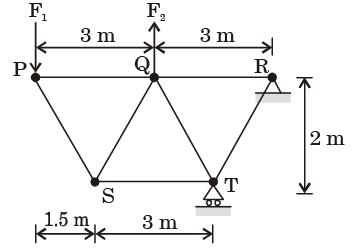Question 6:Two steel truss members, AC md BC, each having cross sectional are of 100 mm2, are subjected to a horizontal force F as shown in figure. All the joints are hinged.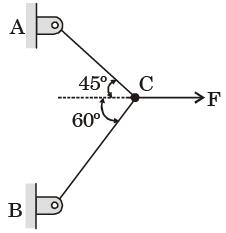The maximum force F in kN that can be applied at C such that the axial stress in any of the truss members DOES NOT exceed 100 MPa is



Question 7:Two steel truss members, AC md BC, each having cross sectional are of 100 mm2, are subjected to a horizontal force F as shown in figure. All the joints are hinged.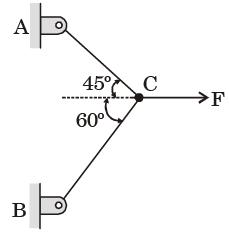If F = 1 kN, the magnitude of the vertical reaction force developed at the point B in kN is



Question 8:Consider a truss PQR loaded at P with aforce Fas shown in the figure.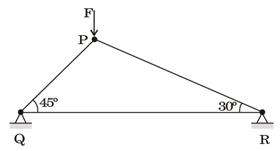The tension in the number QR is



Question 9:The figure shows a pin-jointed plane truss Ioaded at the point M by hanging a mass of 100 kg. The member LN of the truss is subjected to a load of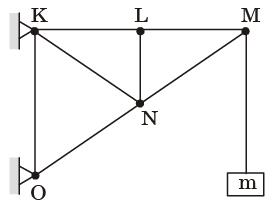Question 10:A truss consists of horizontal members (AC, CD, DB  and EF) and vertical members (CE and DF) having length l each. The-members AE, DE and BF are inclined at 45° to the horizontal For the uniformly distributed load p per unit length on the members EF of the truss shown in figure given below, the force in the member CD is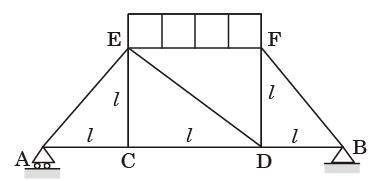Offer running on EduRev: Apply code STAYHOME200 to get INR 200 off on our premium plan EduRev Infinity!

## Engineering Mechanics

37 videos|85 docs|64 tests

,

,

,

,

,

,

,

,

,

,

,

,

,

,

,

,

,

,

,

,

,

;